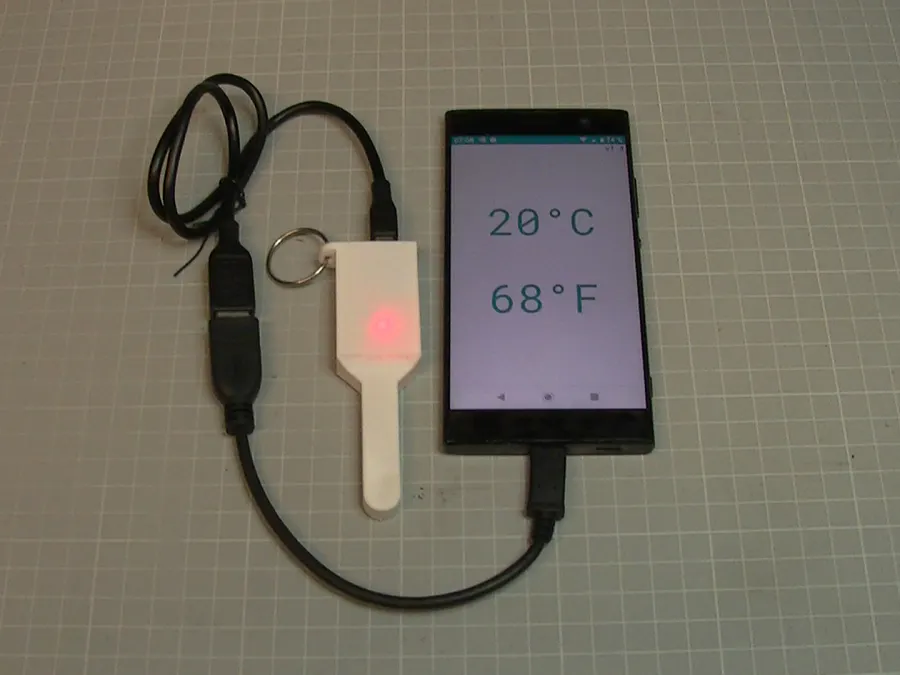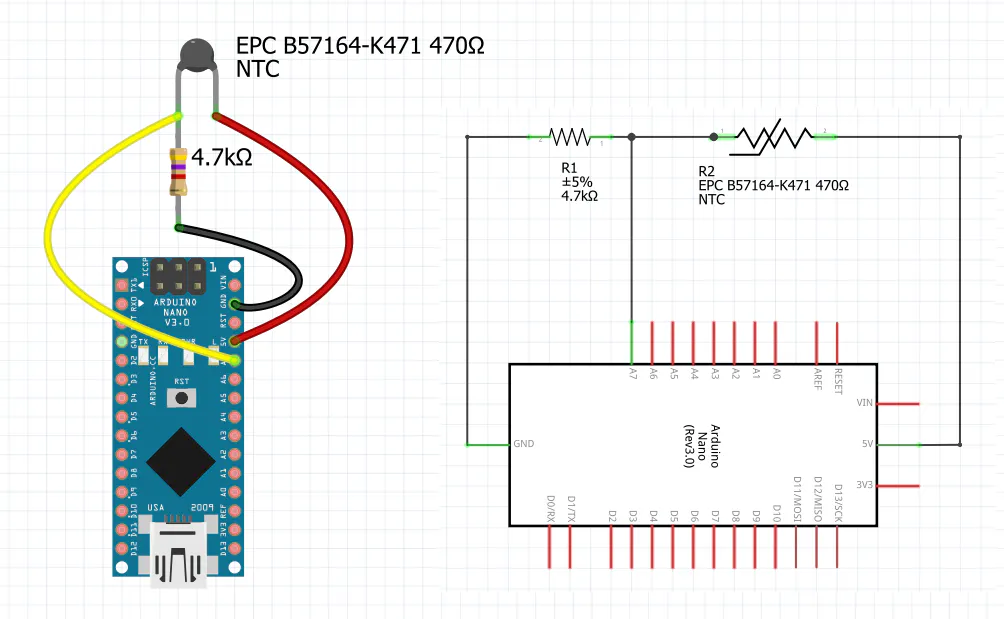Published © Apache-2.0

# Phone Thermometer

A simple and cheap thermometer for your phone or desktop

BeginnerFull instructions provided147## Things used in this project

### Hardware componentsArduino Nano R3
×1
 EPC B57164-K471 470Ω
×1
 4.7 kOhm resistor
×1
 Stranded wire
×1
 OTG cable for your phone
×1

### Software apps and online services

 Github thermo The PWA app for the Chrome browser that displays the temperature in Celsius and Fahrenheit. It is installable and can be used offline if installed.

### Hand tools and fabrication machinesSoldering iron (generic)3D Printer (generic)

Read more

## Schematics

### breadboard and schematic## Code

### Arduino

C/C++
The arduino code for the Nano
```#define R25 470.0f
#define RKNOWN 4700.0f
#define RWIRE 0.2f
#define ARRAY_SIZE 34

// from datasheet
float Array_RT[] = {9573.90f, 7245.52f, 5534.25f, 4262.81f, 3313.36f, 2593.79f, 2047.23f, 1626.62f, 1302.14f, 1048.71f, 850.61f, 693.81f, 569.45f, 470.00f, 390.02f, 325.33f, 272.80f, 229.83f, 194.56f, 165.43f, 141.28f, 121.17f, 104.33f, 90.19f, 78.25f, 68.14f, 59.54f, 52.21f, 45.93f, 40.53f, 35.87f, 31.85f, 28.35f, 25.31f};
float Array_T[] = {-40.0f, -35.0f, -30.0f, -25.0f, -20.0f, -15.0f, -10.0f, -5.0f, 0.0f, 5.0f, 10.0f, 15.0f, 20.0f, 25.0f, 30.0f, 35.0f, 40.0f, 45.0f, 50.0f, 55.0f, 60.0f, 65.0f, 70.0f, 75.0f, 80.0f, 85.0f, 90.0f, 95.0f, 100.0f, 105.0f, 110.0f, 115.0f, 120.0f, 125.0f};

int a7;

float RT;
float T = -273.15;

int idx1, idx2;

void setup() {
Serial.begin(19200);

pinMode(LED_BUILTIN, OUTPUT);

// blink fast to indicate startup / reset
for (int i=0; i<5; i++){
digitalWrite(LED_BUILTIN, HIGH);
delay(50);
digitalWrite(LED_BUILTIN, LOW);
delay(50);
}
}

void loop() {
digitalWrite(LED_BUILTIN, HIGH);
delay(1000);

a7 = analogRead(7); // 10 bit => a7  [0..1023]

// example 2 bit  : a7  [0..3]    => 0 == 0 * 5/3 V,    1 == 1 * 5/3V , 2 == 2 * 5/3 V, 3 == 3 * 5/3 V
// example 10 bit : a7  [0..1023] => 0 == 0 * 5/1023 V, 1 == 1 * 5/1023 V, ..., 1023 == 1023 * 5/1023 V

// https://en.wikipedia.org/wiki/Voltage_divider (Resistive divider)
// a7 = 1023 * RKNOWN / (RT + RKNOWN)
// => (RT + RKNOWN) = 1023 * RKNOWN / a7
// => RT = 1023 * RKNOWN / a7 - RKNOWN
// => RT = RKNOWN * (1023 / a7 - 1)

RT = RKNOWN * (1023.0f / (float)a7 - 1.0f) + RWIRE;

for (int i=1; i < ARRAY_SIZE; i++) {
if (Array_RT[i] < RT){
idx1 = i - 1;
idx2 = i;
break;
}
}

T = (Array_T[idx2] - Array_T[idx1]) / (Array_RT[idx2] - Array_RT[idx1]) * (RT - Array_RT[idx1]) + Array_T[idx1];

/*Serial.print("a7: "); Serial.print(a7); Serial.print(" ");
Serial.print("RT: "); Serial.print(RT); Serial.print(" ");
Serial.print("idx1: "); Serial.print(idx1); Serial.print(" ");
Serial.print("idx2: "); Serial.print(idx2); Serial.print(" ");
Serial.print("Array_RT[idx1]: "); Serial.print(Array_RT[idx1]); Serial.print(" ");
Serial.print("Array_RT[idx2]: "); Serial.print(Array_RT[idx2]); Serial.print(" ");
Serial.print("Array_T[idx1]: "); Serial.print(Array_T[idx1]); Serial.print(" ");
Serial.print("Array_T[idx2]: "); Serial.print(Array_T[idx2]); Serial.print(" ");
Serial.print("T: "); Serial.print(T); Serial.print(" ");
Serial.print("round(T): "); Serial.print(round(T)); Serial.print(" ");*/
Serial.println(round(T));

digitalWrite(LED_BUILTIN, LOW);
delay(1000);

}
```

### Github

The Project/Repository on Github

## Credits

### AJBauer

0 projects • 0 followers